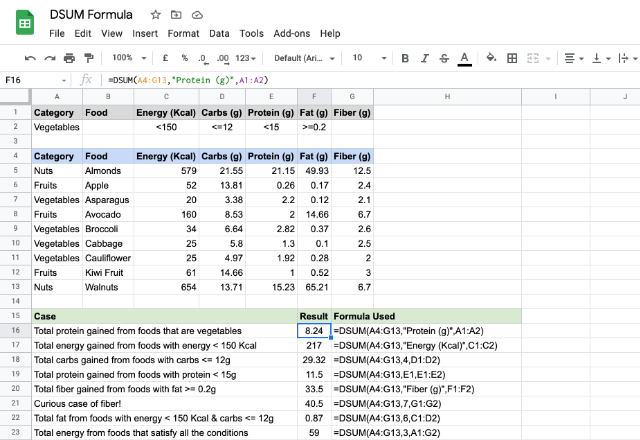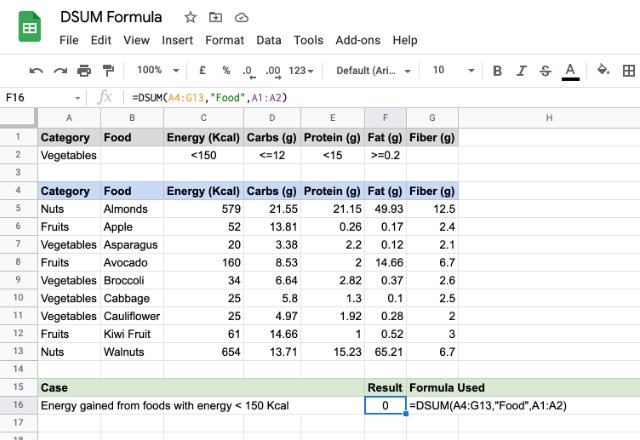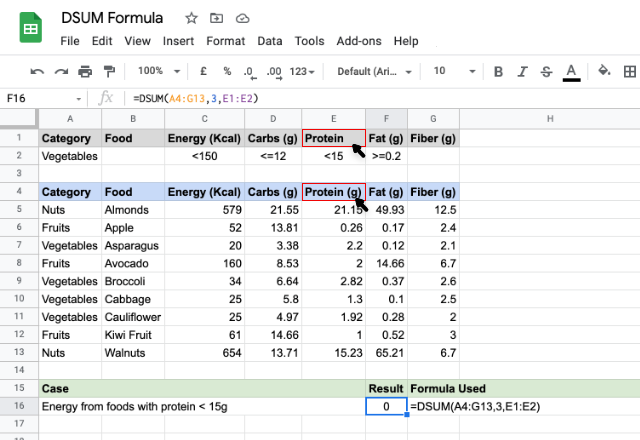# How to use the DSUM Google Sheets formulaThe DSUM Google Sheets formula is almost similar to that of the SUM formula, but with a distinction. It gives us the sum of values available in a table like range, that meet a specified criteria. This is analogous to an SQL database sum query. The ‘D’ in the DSUM stands for ‘Database’, therefore, we can call this a Database SUM formula.

### Syntax

DSUM(database, field, criteria)

• database – is the reference to a structured data range that consists of labels, for each column, in the first row.
• field – indicates the column on which the DSUM formula should sum the numeric values. This can be a text or a column index too.
• criteria – this is a reference to a range that consists of criteria that the formula uses to filter the database values before counting.

### Usage: DSUM Google Sheets formula

Let us assume that the below list of foods is our secret diet suggested by a nutritionist. We will try the formula out using a few examples.We see that the data set is in a labeled tabular form spanning across cells A4 through to G13. And the criteria is keyed in the cells A1 through to G2.

Please note from the cases illustrated above that we can specify one or more criteria to filter the summing process. For example, the case in the row # 7 sums the values in the “Carbs (g)” column, subject to the criteria in the cell D2. Whereas the case in row # 11 sums the values from “Fat (g)” column, subject to the criteria within the cells C2 and D2. The last case on row # 12 includes all the criteria from A2 through to G2, and apparently there are two rows (9 & 10) that satisfies all of these conditions, hence the result 59.

The case on the row # 10 is a rather interesting one! We didn’t specify anything in the cell G2, yet, we gave that reference to the DSUM formula. The result is 40.5, because it summed everything in the “Fiber (g)” column as there is no criteria to filter out the rows.

##### Unwarranted Cases

Now, let us talk about pitfalls. Here is the first one, which we might already be aware of. Please consider the first case in the snapshot below.Here, we tried summing non numeric values! Just like the SUM formula, DSUM formula sums only numbers. Therefore, it returned ‘0’ as output.

Here’s the second one in the image below.Seemingly there’s nothing wrong with the formula this time but the result isn’t what we are expecting. The problem lies within the labels. Apparently, a missing or a mismatched label doesn’t help the DSUM formula, as its working hinges on the field name that the second parameter takes. Since the labels didn’t match, it returned the sum as zero.